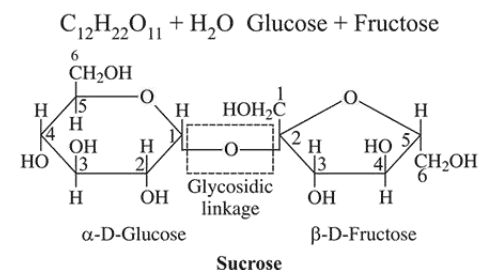# Which of the following statements is not true about sucrose?Question:

Which of the following statements is not true about sucrose?

1. It is a non reducing sugar.

2. The glycosidic linkage is present between $\mathrm{C}_{1}$ of $\alpha$-glucose and $\mathrm{C}_{1}$ of $\beta$-fructose.

3. It is also named as invert sugar.

4. On hydrolysis, it produces glucose and fructose.

Correct Option: , 3

Solution:

Sucrose contains glycosidic link between $C_{1}$ of $\alpha-D$ glucose and $C_{2}$ of $\beta$-D-fructose.